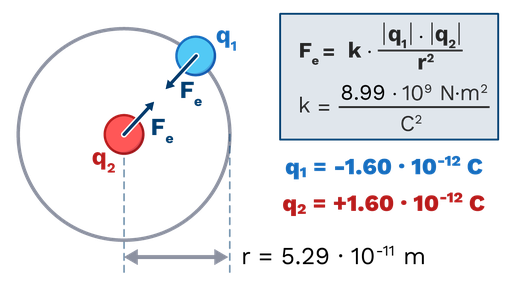In this example, we will use Coulomb’s law to determine the electrostatic force between a proton and an electron in a very simplified classical model of the hydrogen atom.

First, we will determine the magnitude of the force using the equation of Coulomb’s law. Then, we will determine the direction of the force, using the part of Coulomb’s law that states that particles with opposite charges attract and particles with like charges repel.

The electrostatic force between two particles depends on:

• Constant of Coulomb’s law (k): This is simply a constant of proportionality, it is always the same every time we use Coulomb’s law. Its value in the SI is 8.99·109 Nm2/C2.
• Charge of the first particle (q1): We can take this as the electron. The charge of the electron in the SI is 1.60·10-19C and it is negative in sign.
• Change of the second particle (q2): We can take this as the proton. The charge of the proton in the SI is 1.60·10-19C and it is positive in sign. Notice that it is the same quantity of charge as the electron, but it has a different sign.
• Distance between the particles (r): We will take this to be the radius of the hydrogen atom, which is 5.29·10-11m.

The equation of Coulomb’s law is:

Fe= k |q1|·|q2|/r2.

We substitute the values of the data we have gathered in the equation:

Fe=(8.99·109 Nm2/C2)·(1.60·10-19C)· (1.60·10-19C)/(5.29·10-11m)2=8.22·10-8N

This is the magnitude of the electrostatic force.

Now, we must determine its direction. The force acts along the line that joins the two particles, but it could be an attractive force (which pulls them together) or a repulsive force (that drives them apart). The electron and the proton have charges of opposite signs. Because opposite charges attract, the force pulls the electron and the proton together, acting in the line that joins them. That is the direction of the electrostatic force.Figure: Simplified version of the hydrogen atom. The arrows point in the direction of the electrostatic forces in this situation.